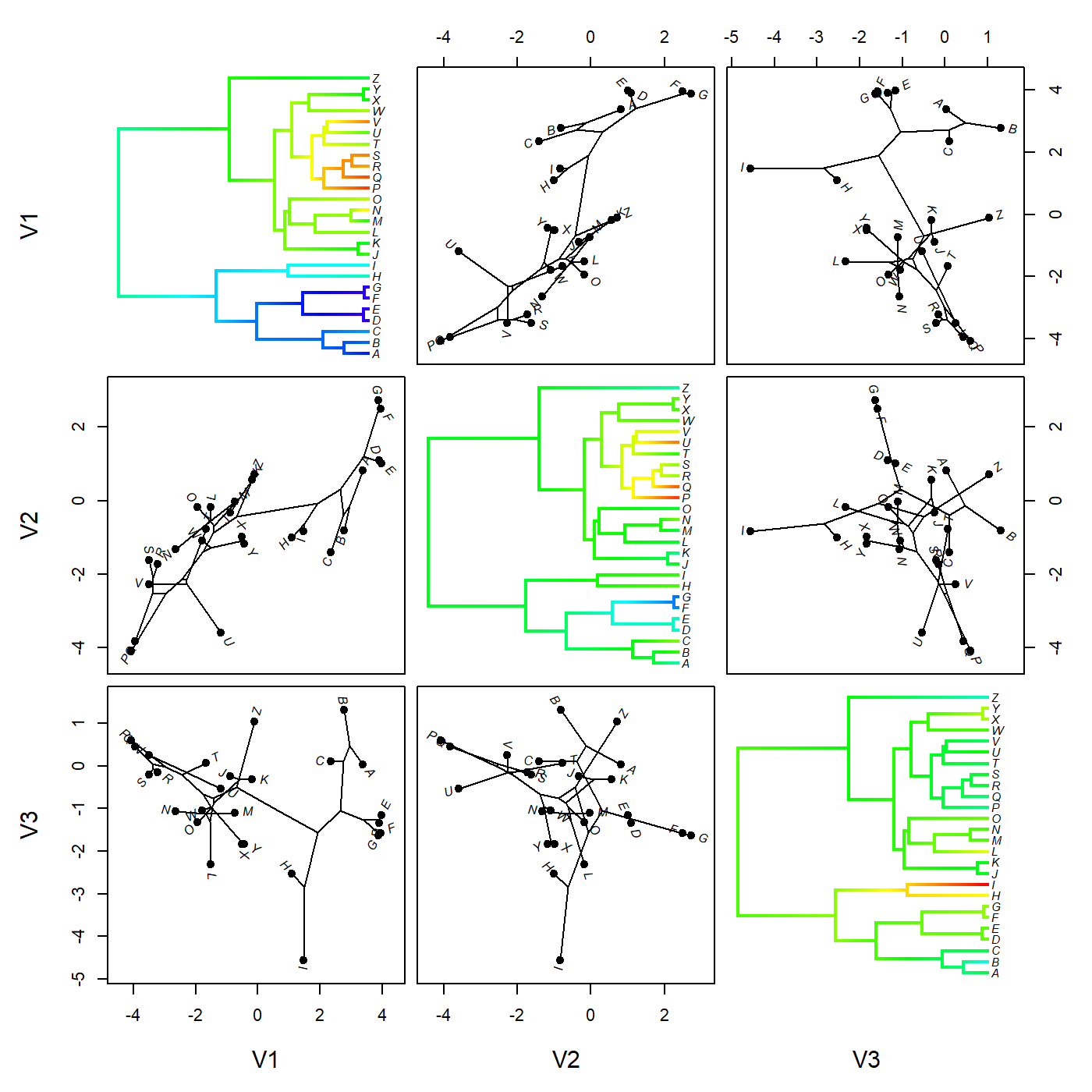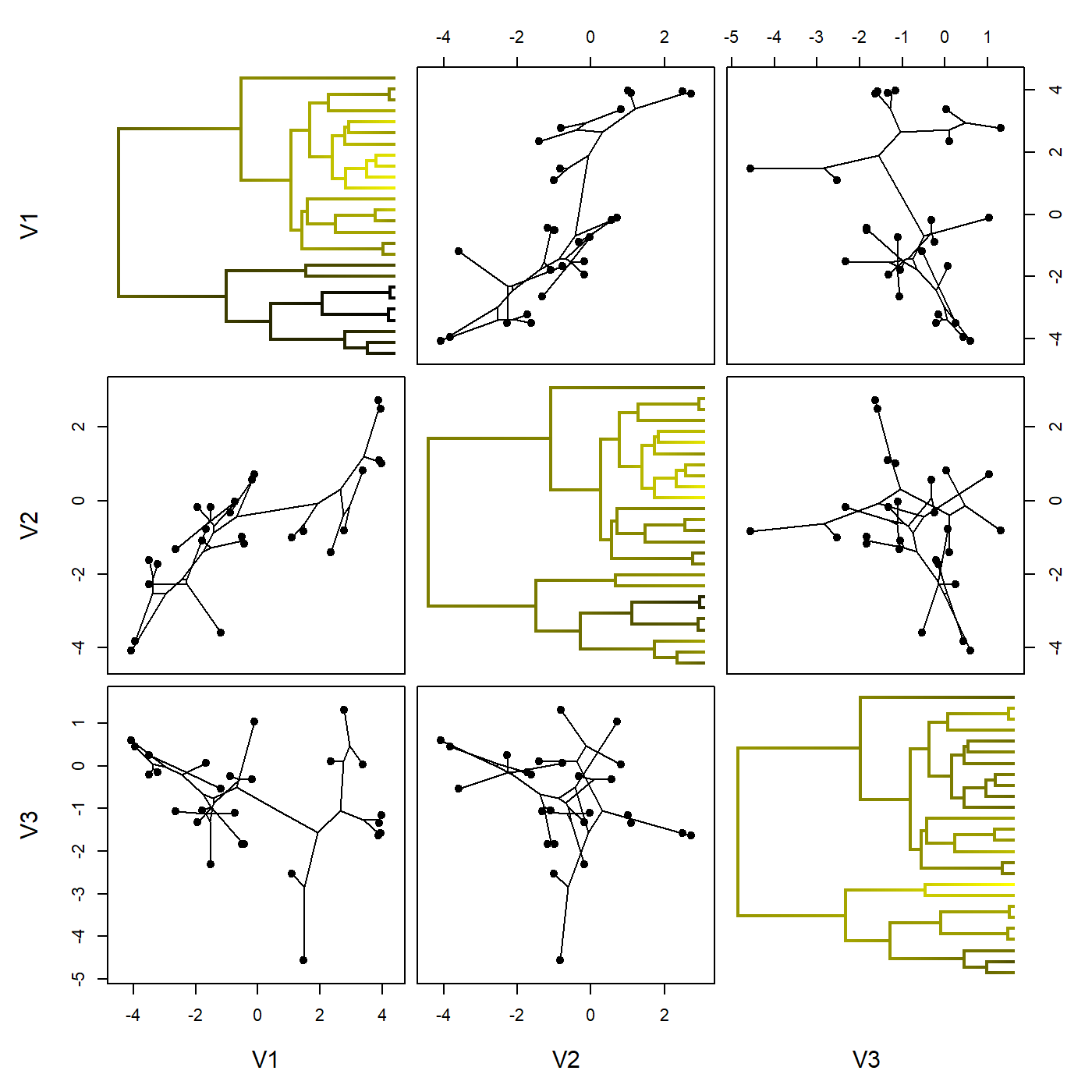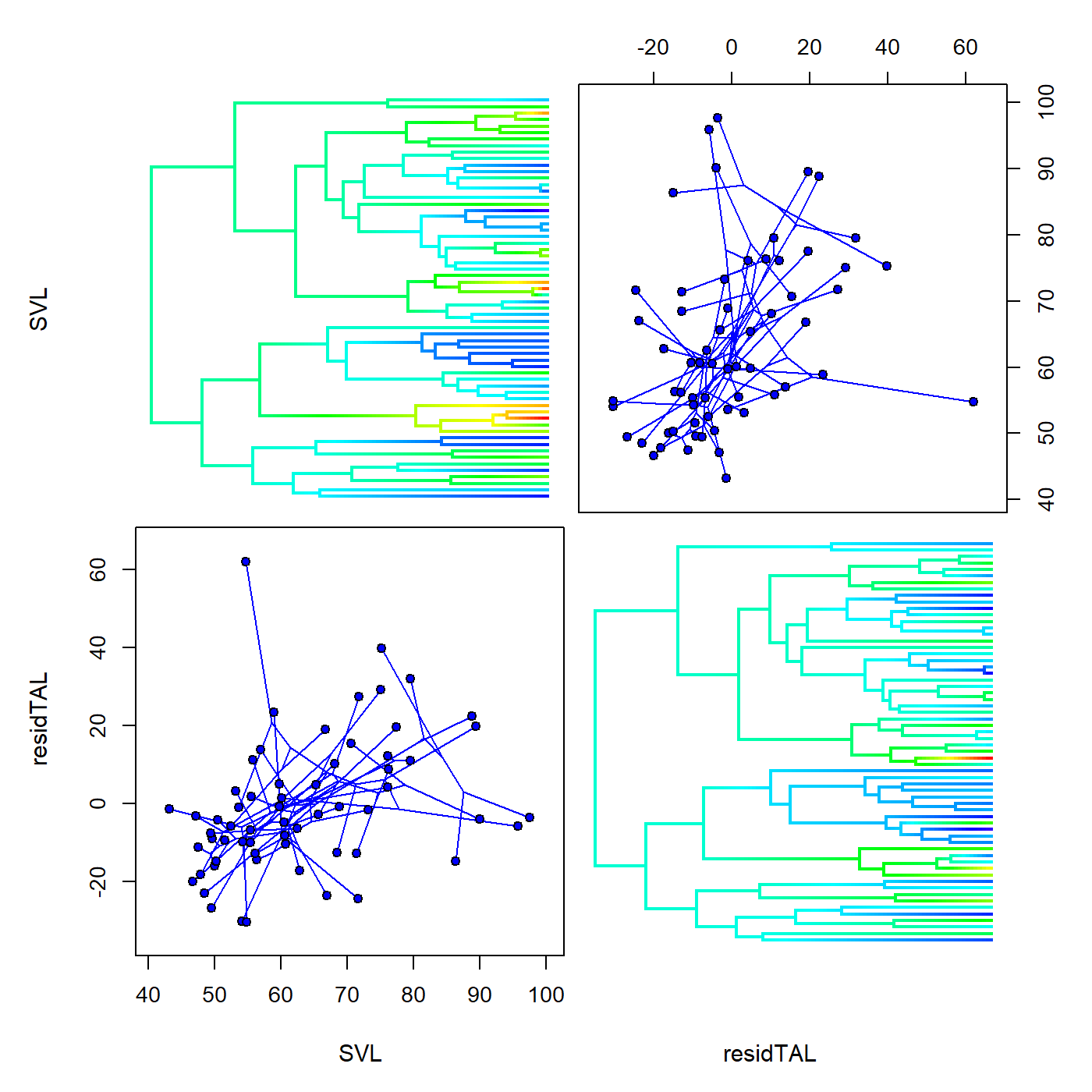## Friday, June 7, 2019

### The phylogenetic multivariate scattergram for continuous traits

I just pushed another couple of new updates to phytools (1, 2).

First, I added two new functions to the namespace, `phenogram95` and `phyloScattergram`. These previously existed in the package but as options within the plotting function `fancyTree`. The `phenogram95` method is quite popular, but the 'phylogenetic scattergram' I'd yet to see used in publication. I have also added some additionally flexibility to the latter function which perhaps might help it see some more use.

Second, I created a new S3 method called `setMap`. On `"densityMap"` and `"contMap"` objects this should work exactly the same as the old `setMap` function, but it will also work for the `"phyloScattergram"` object without having to call a differently named function.

Here's a demo of the 'new' `phyloScattergram` function & `setMap` S3 method.

First, with some simulated data:

``````library(phytools)
tree<-pbtree(n=26,tip.label=LETTERS)
V<-matrix(c(
1.0,0.7,0.0,
0.7,1.4,0.0,
0.0,0.0,0.5),3,3)
X<-sim.corrs(tree,V)
object<-phyloScattergram(tree,X,fixed.lims=TRUE)
``````
``````## Computing multidimensional phylogenetic scatterplot matrix...
````````````object
``````
``````##
## Object of class "phyloScattergram" for 3 continuous traits.
## To replot enter "plot(object_name)" at the prompt.
``````
``````object<-setMap(object,c("yellow","black"))
plot(object,ftype="off")
``````Next, here are some real data that I pulled off of Dryad:

``````liolaemus<-read.tree(file=
"pympal","pympun"))
row.names=1)
liol.data<-iguanids[liolaemus\$tip.label,c(1,3)]
liol.data\$TAL<-as.numeric(liol.data\$TAL)
liol.data\$residTAL<-
phyl.resid(liolaemus,x=as.matrix(liol.data[,1,
drop=FALSE]),Y=as.matrix(liol.data[,2,
drop=FALSE]))\$resid
liolaemus<-paintSubTree(liolaemus,
Ntip(liolaemus)+1,"1")
object<-phyloScattergram(liolaemus,
liol.data[,c(1,3)],plot=FALSE)
``````
``````## Computing multidimensional phylogenetic scatterplot matrix...
``````
``````object<-setMap(object,invert=TRUE)
plot(object,colors=setNames("blue",1),
ftype="off")
``````Neat.